﻿ 舰船消磁实验载具的设计与分析
 舰船科学技术2019, Vol. 41Issue (4): 119-123PDF

1. 江苏科技大学 机械工程学院，江苏 镇江 212003;
2. 江苏科技大学 能源与动力工程学院，江苏 镇江 212003

Design and analysis of the carrier for ship degaussing experiment
SHE Jian-guo1, CHEN Yang1, GE Jian-fei1, CHEN Ning2
1. School of Mechanical Engineering, Jiangsu University of Science and Technology, Zhenjiang 212003, China;
2. School of Energy and Power Engineering, Jiangsu University of Science and Technology, Zhenjiang 212003, China
Abstract: In order to overcome the shortcomings of traditional degaussing experiment, to realize the dynamic magnetic measurement process, to improve the efficiency of gathering data, the author puts forward a carrier for ship degaussing experiment. In this paper, according to the relationship between the induction magnetic field and ship heading and posture change, the author completes the overall structural design, and its characteristic of dynamics is researched. The results reflect the real status about force and motion of the carrier, and verifies working characteristic of the carrier, which meets requirements of ship degaussing experiment.
Key words: ship degaussing experiment     carrier     structural design     dynamics
0 引　言

1 运动功能分析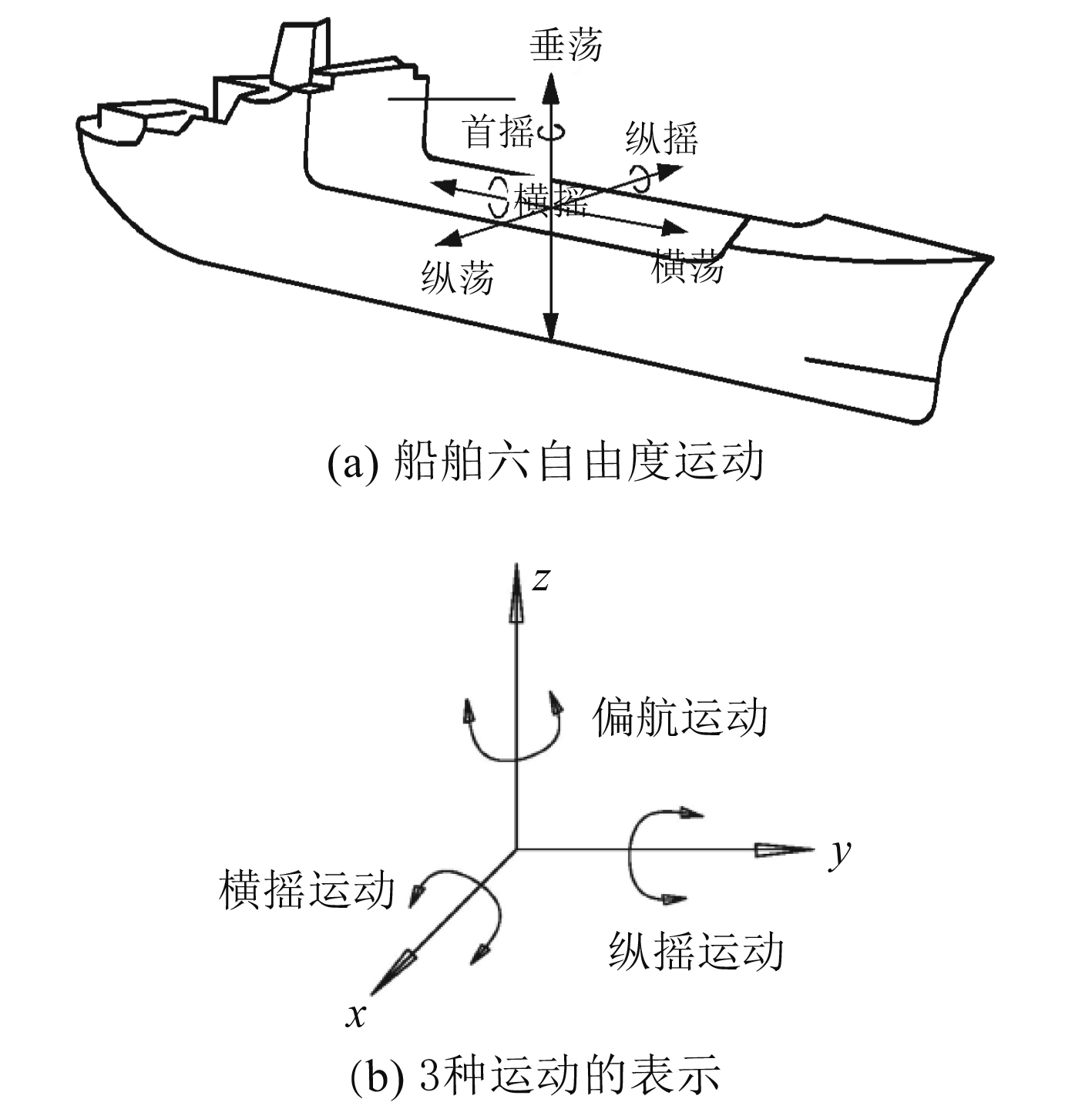图 1 各种运动在坐标系中的表示 Fig. 1 Representation of various motion in coordinate表 1 基本运动类型与运动范围 Tab.1 The type and range of motion
2 结构设计 2.1 结构设计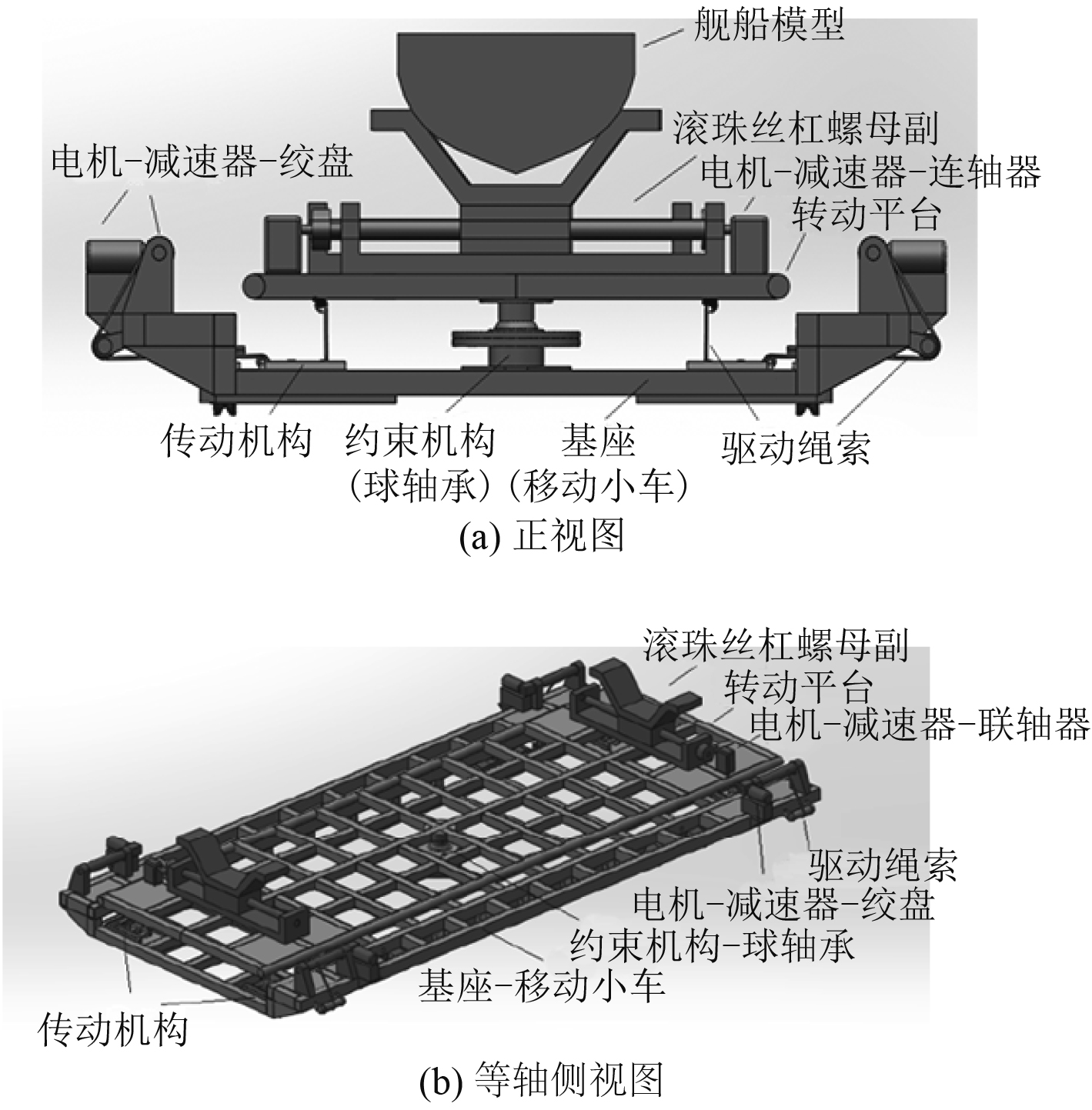图 2 载具的三维模型 Fig. 2 3D model of the carrier
2.2 自由度分析

 $F = 6(n - g - 1) + \sum\limits_{i = 1}^g {{f_i}} \text{。}$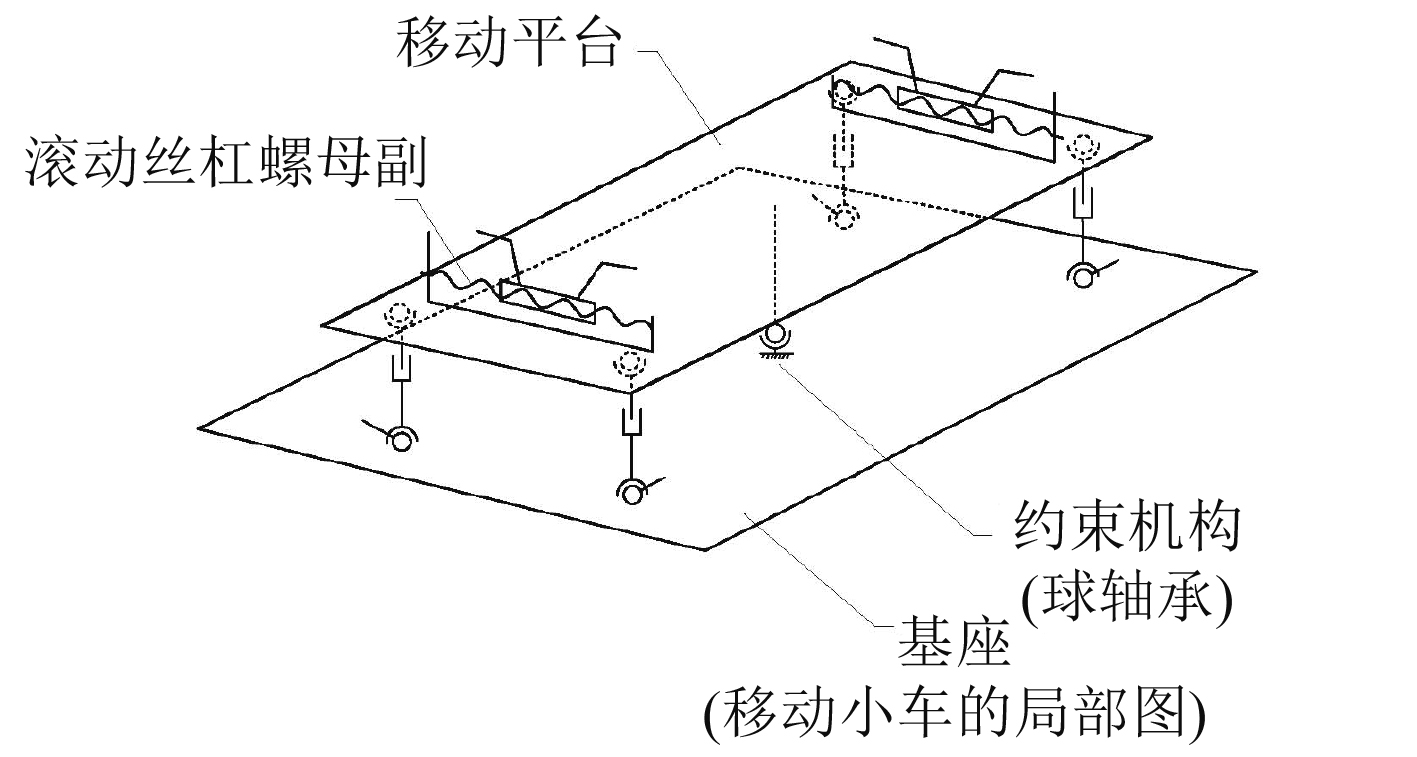图 3 等效机构简图 Fig. 3 Simplified diagram of equivalent mechanism

 $F = 6(10 - 13 - 1) + 27 = 3\text{。}$

3 动力学分析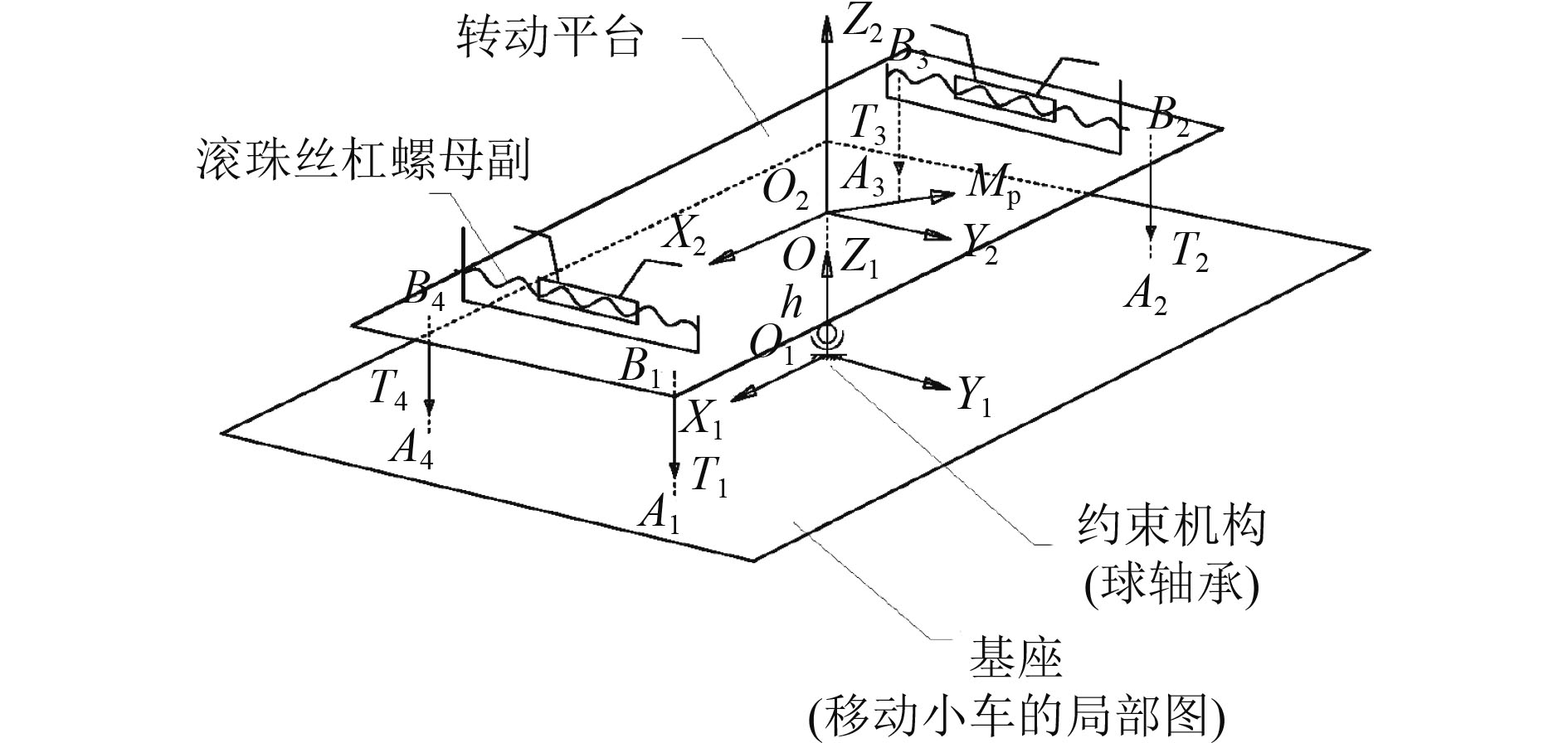图 4 载具转动平台受力简图 Fig. 4 Stress diagram of the rotating platform of the carrier

 $\left\{ {\begin{array}{*{20}{c}} {\displaystyle\sum\limits_{i = 1}^4 {{{{T}}_i} + {{F}} + {{Q}} + m{{g}} + {{{F}}_c} = 0} }\text{，}\\ {\displaystyle\sum\limits_{i = 1}^4 {{}^1{{{R}}_2}{}^2{{{b}}_i} \times {{{T}}_i} + {{{M}}_p} + {{{M}}_c} = 0} }\text{。} \end{array}} \right.$ (1)

 ${{{F}}_c}=m{{v}}_c^{'}\text{，}$ (2)
 ${{{M}}_c} = - {{{I}}_c}{}^1{{\omega }}_2^{'} - {}^1{{{\omega }}_2} \times ({{{I}}_c}{}^1{{{\omega }}_2})\text{。}$ (3)

 ${{UV}} = {{W}}\text{，}$ (4)

 ${{W}} = {\left[ {\begin{array}{*{20}{c}} {{{{W}}_1}}&{{{{W}}_2}} \end{array}} \right]^{\rm T}} = \left[ {\begin{array}{*{20}{c}} { - ({{F}} + {{Q}} + m{{g}} + {{{F}}_c})}\\ { - ({{{M}}_p} + {{{M}}_c})} \end{array}} \right]\text{。}$ (5)

4 动力学仿真 4.1 机构的运动参数表 2 机构的运动参数 Tab.2 Motion parameters of the mechanism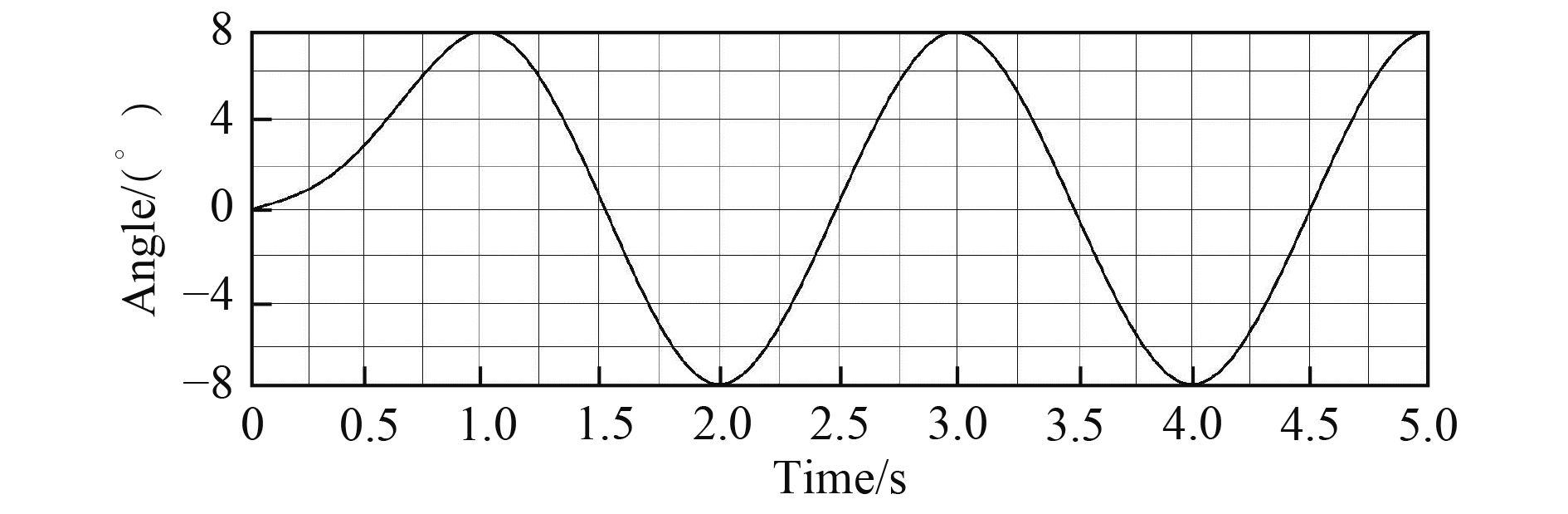图 5 转动平台做横摇运动时的曲线 Fig. 5 The curve of rotating platform doing rolling motion
4.2 动力学仿真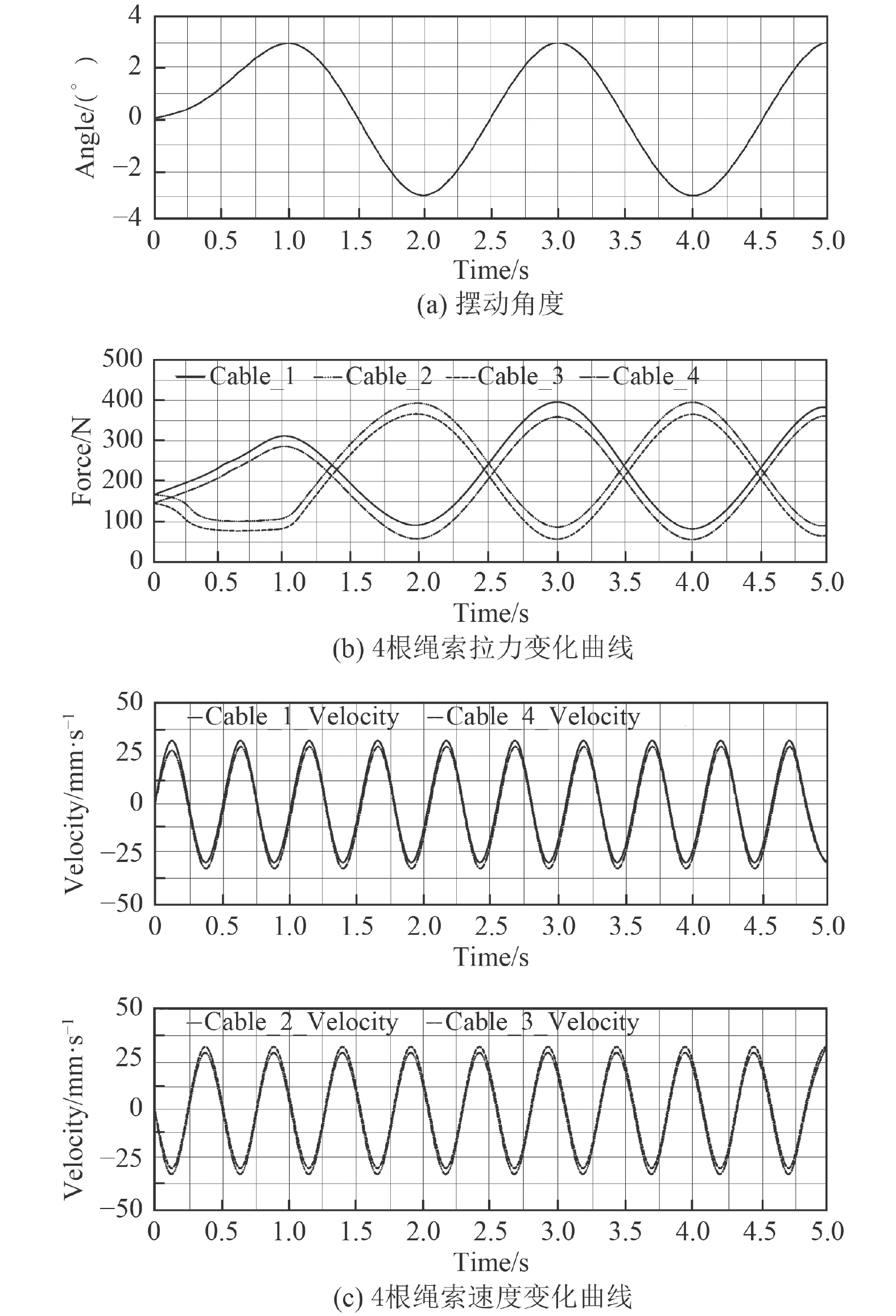图 6 转动平台做横摇运动时仿真曲线 Fig. 6 Simulation curve of rotating platform carried out rolling motion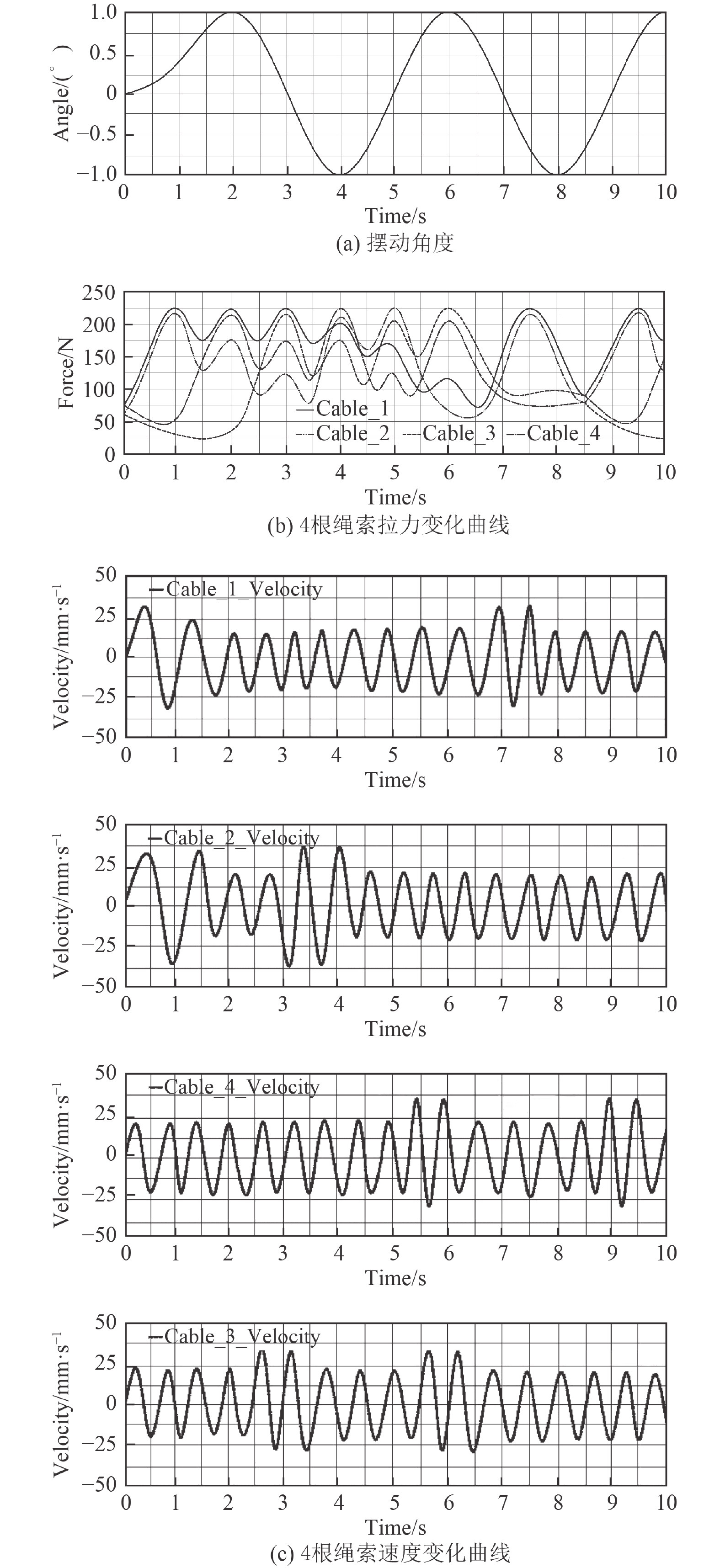图 9 转动平台做联动运动时仿真曲线 Fig. 9 Simulation curve of rotating platform doing coupling motion

1）从图6可以看出，当转动平台携带舰船模型做横摇运动时，初始时保持静止状态，转动平台摆角与速度值均为0，而绳索拉力值不为0，主要是为了保证转动平台平衡，绳索需处于张紧状态，所以初始时拉力保持定值。

2）从图7可以看出，当转动平台携带舰船模型做纵摇运动时，初始时保持静止状态，转动平台摆角与速度的值均为0；为了保证转动平台平衡，绳索需处于张紧状态，故各根绳索拉力值不为0。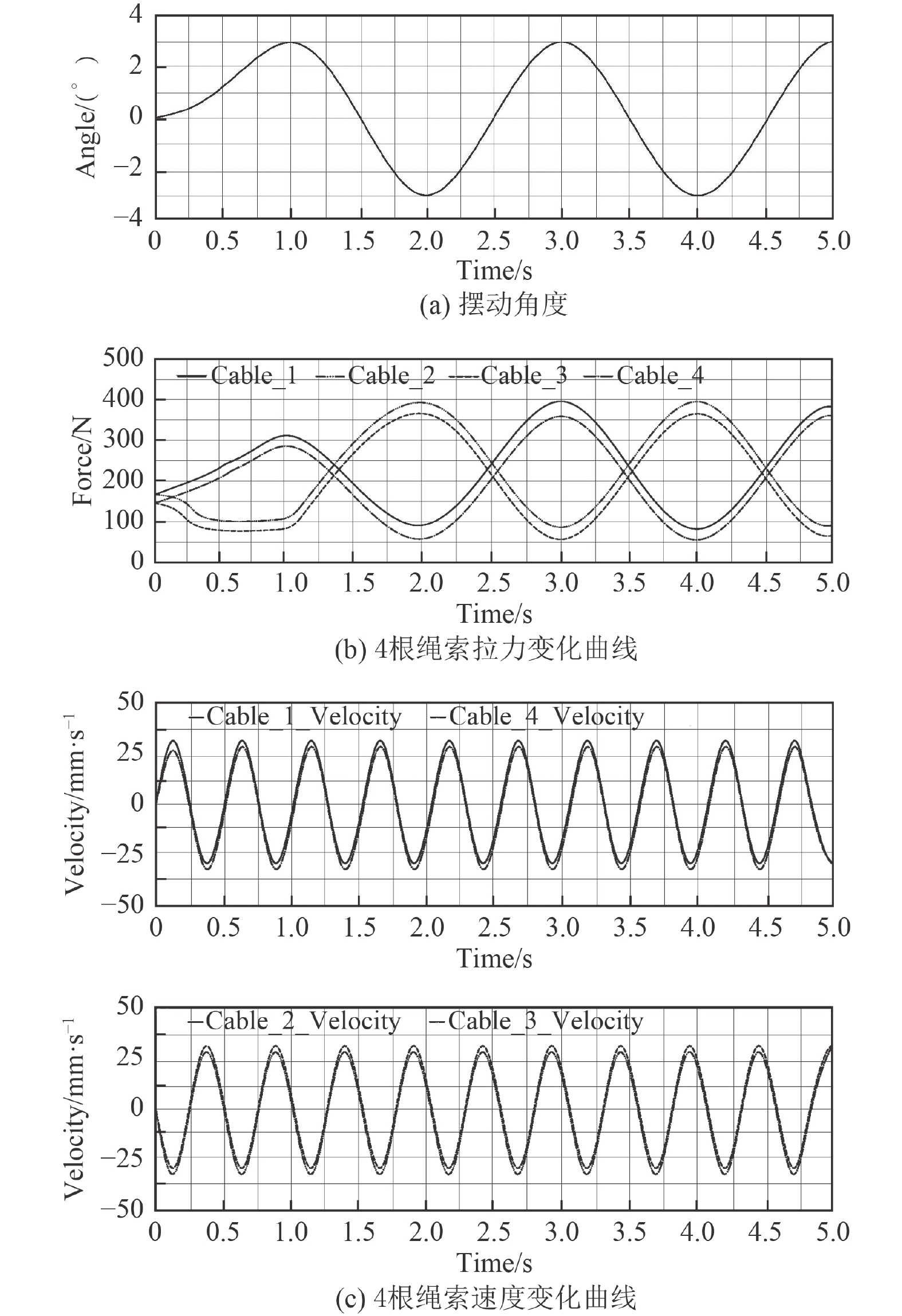图 7 转动平台做纵摇运动时仿真曲线 Fig. 7 Simulation curve of rotating platform doing pitching motion

3）从图8可以看出，当转动平台携带舰船模型做偏航运动时，初始时保持静止状态，转动平台摆角与速度的值均为0；为保证转动平台平衡，绳索需处于张紧状态，故绳索拉力值不为0。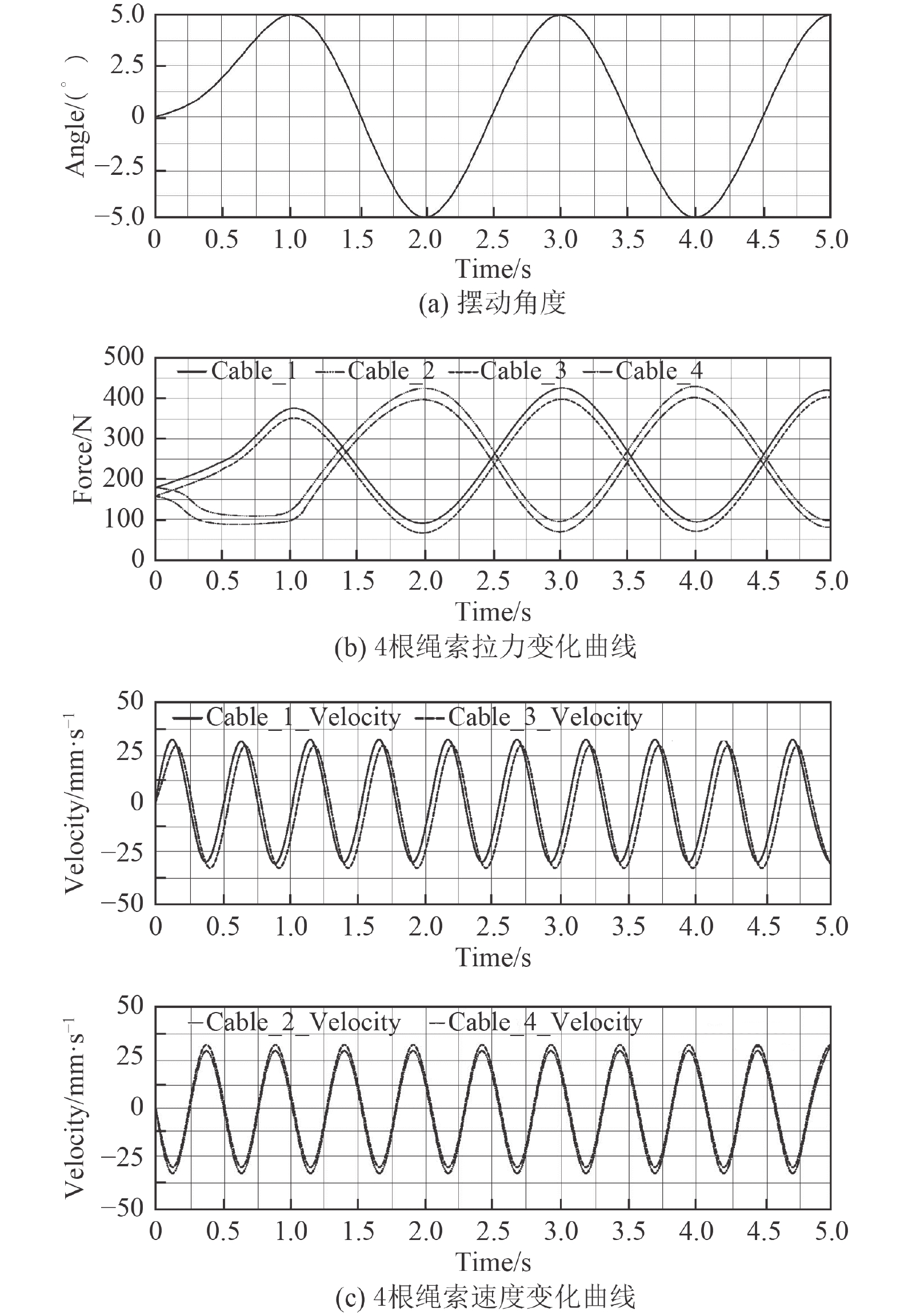图 8 转动平台做偏航运动时仿真曲线 Fig. 8 Simulation curve of rotating platform doing yawing motion

4）从图9可以看出，当转动平台携带舰船模型做联动运动时，初始时保持静止状态，转动平台摆角与速度值均为0，绳索拉力值不为0。

5 结语

  刘胜道, 王巍, 肖存龙. 低磁钢舰艇消磁系统绕组安匝调整[J]. 船电技术, 2013, 33(4): 25-27. DOI:10.3969/j.issn.1003-4862.2013.04.008  冯铁城, 朱文蔚, 顾树华. 船舶操纵与摇荡(修订本)[M]. 国防工业出版社, 1989.  张立勋, 刘攀, 王克义, 等. 绳索牵引并联康复机器人的建模与控制[J]. 哈尔滨工程大学学报, 2009, 30(1): 81-85. DOI:10.3969/j.issn.1006-7043.2009.01.014  严传续. 小型船舶运动模拟器研究[D]. 哈尔滨: 哈尔滨工程大学, 2008.  禹润田. 新型绳驱动并联踝关节康复机构设计及分析[D]. 北京: 北京交通大学, 2015. 3.  陈立平. 机械系统动力学分析及ADAMS应用教程[M]. 北京: 清华大学出版社, 2005.